# Use elementary row operations to reduce the given matrix to row echelon form and reduced row...

Use elementary row operations to reduce the given matrix to row echelon form and reduced row echelon form. Please note when it hits REF and RREF. Thank you!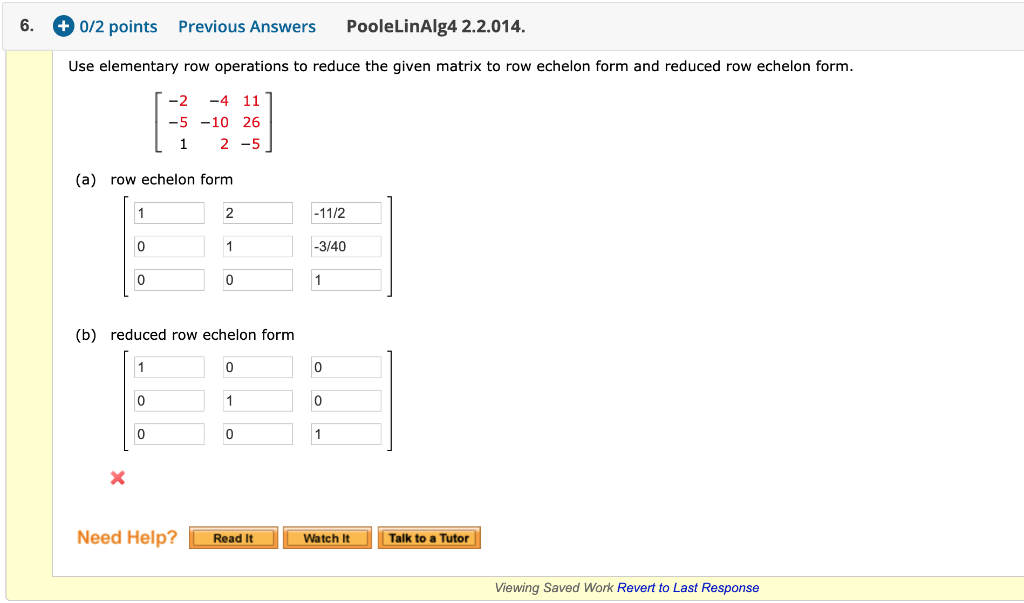6. + 0/2 points Previous Answers PooleLinAlg4 2.2.014. Use elementary row operations to reduce the given matrix to row echelon form and reduced row echelon form. [-2 -4 11 | -5 -10 26 Li 2 -5] (a) row echelon form 2 1 -1172 -3/40 0 1 (b) reduced row echelon form 0 1 0 Need Help? Read It Watch it Talk to a Tutor Viewing Saved Work Revert to Last Response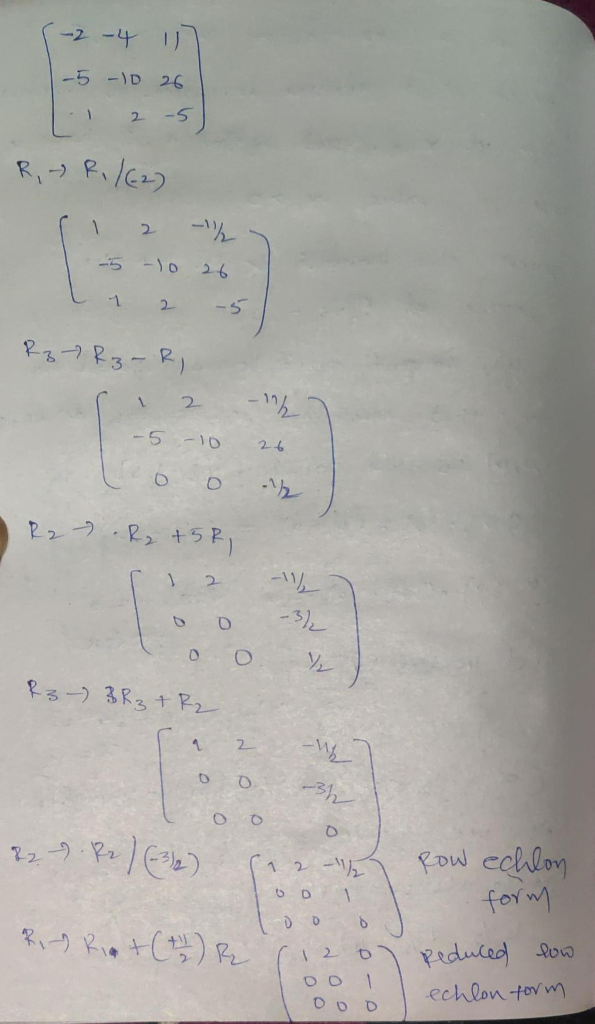If you have any questions please let me know

#### Earn Coin

Coins can be redeemed for fabulous gifts.

Similar Homework Help Questions
• ### 4. Give the row-echelon form and the reduced row-echelon form of the matrix: A = 11...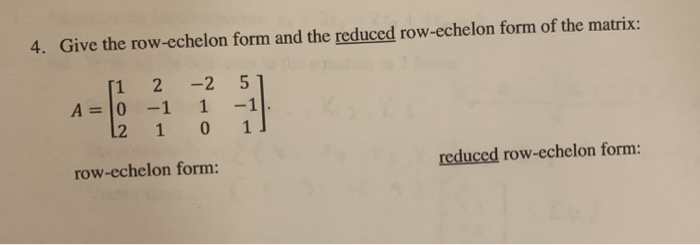4. Give the row-echelon form and the reduced row-echelon form of the matrix: A = 11 2 0 -1 12 1 -2 51 1 -1 0 1] row-echelon form: reduced row-echelon form:

• ### Problem 12 In Exercises 1 - 2 row reduce the given matrix to reduced echelon form...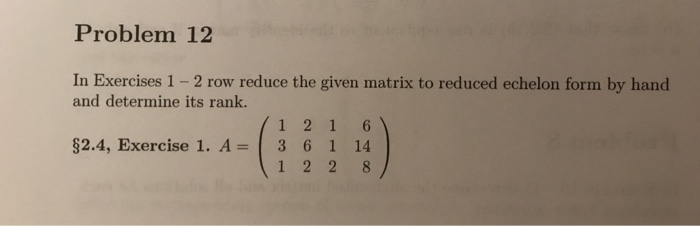Problem 12 In Exercises 1 - 2 row reduce the given matrix to reduced echelon form by hand and determine its rank. 1 1 2 1 6 ) \$2.4, Exercise 1. A = 3 6 1 14 1 1 2 2 8

• ### Use Gaussian elimination to find a row echelon form (not reduced row echelon form) of the...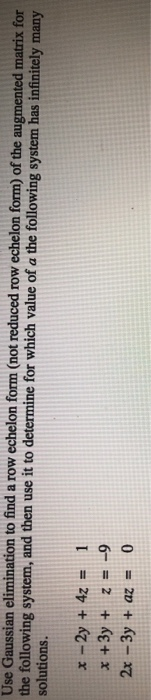Use Gaussian elimination to find a row echelon form (not reduced row echelon form) of the augmented matrix for the following system, and then use it to determine for which value of a the following system has infinitely many solutions. x - 2y + 4z = 1 * +3y + z = -9 2x - 3y + az = 0

• ### (5 points) The following augmented matrix is in reduced row echelon form. Decode from the matrix...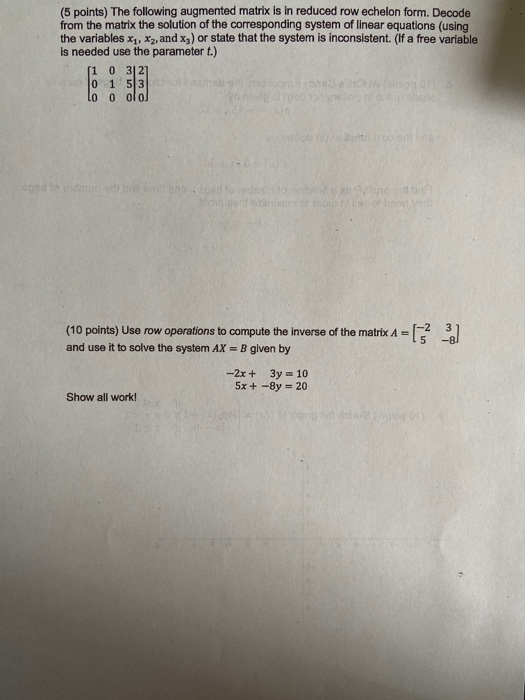(5 points) The following augmented matrix is in reduced row echelon form. Decode from the matrix the solution of the corresponding system of linear equations (using the variables X1, X2, and x3) or state that the system is inconsistent. (if a free variable is needed use the parameter t.) 1 0 3121 0 1 53 Lo 0 olo) con (10 points) Use row operations to compute the inverse of the matrix A = [ 53 -2] and use it to...

• ### Use row-reduction to put the following matrix to reduced row echelon form. 1 5 4 2...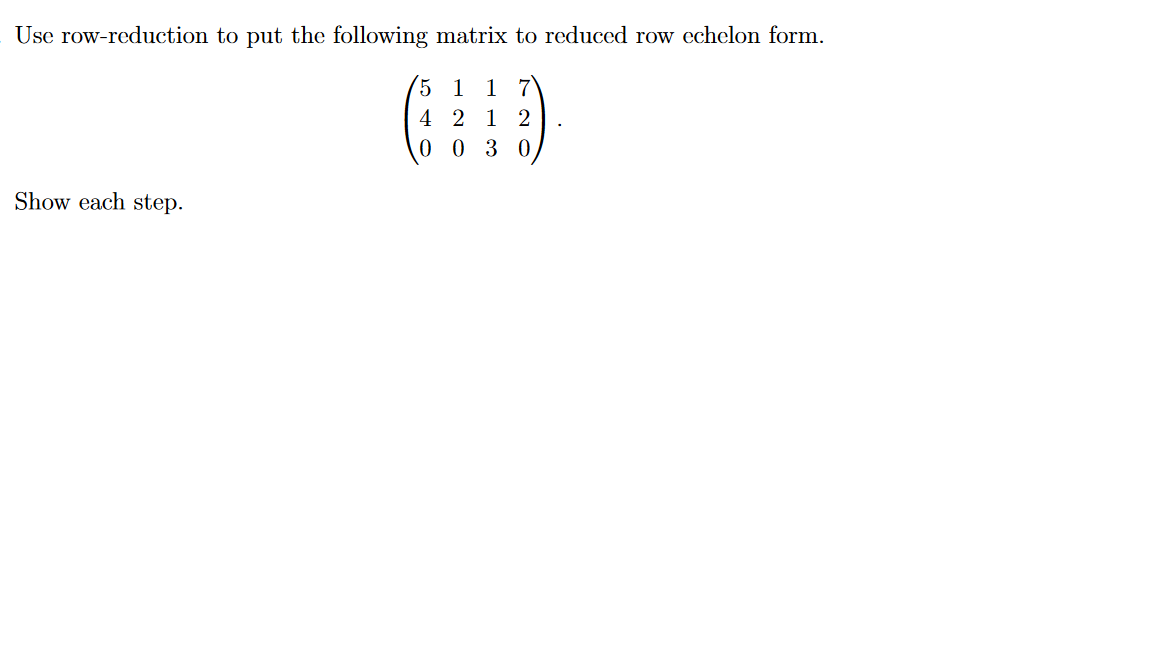Use row-reduction to put the following matrix to reduced row echelon form. 1 5 4 2 1 2 0 0 3 0 Show each step.

• ### Given the following system of linear equations 1. 2xi + 4x2 + 8 x3 + x. +2x,3 a) Write the augmented matrix that represents the system b) Find a reduced row echelon form (RREF) matrix that is row...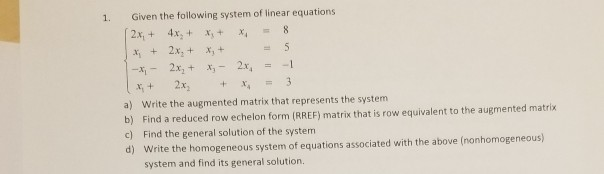Given the following system of linear equations 1. 2xi + 4x2 + 8 x3 + x. +2x,3 a) Write the augmented matrix that represents the system b) Find a reduced row echelon form (RREF) matrix that is row equivalent to the augmented matrix c) Find the general solution of the system d) Write the homogeneous system of equations associated with the above (nonhomogeneous) system and find its general solution. Given the following system of linear equations 1. 2xi + 4x2...

• ### Question 3 Use row-reduction to put the following matrix to reduced row echelon form. 5 1...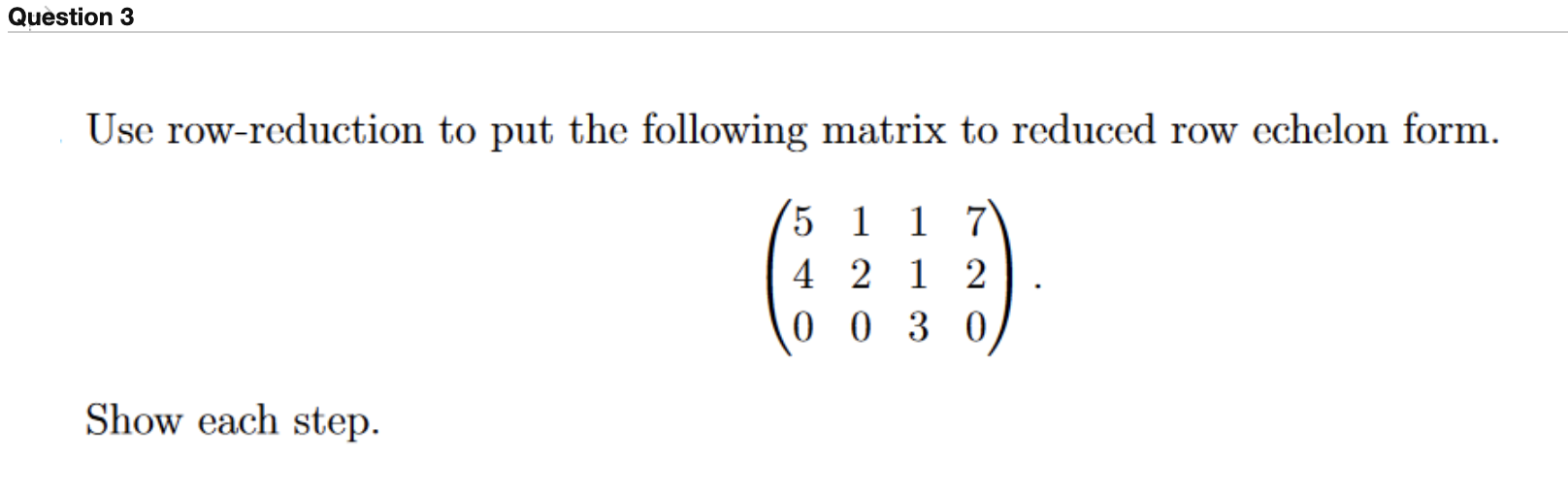Question 3 Use row-reduction to put the following matrix to reduced row echelon form. 5 1 1 7 4 2 1 2 0 0 3 0 Show each step.

• ### 4. (a) Row reduce the matrices A and B below to reduced row echelon form (RREF)....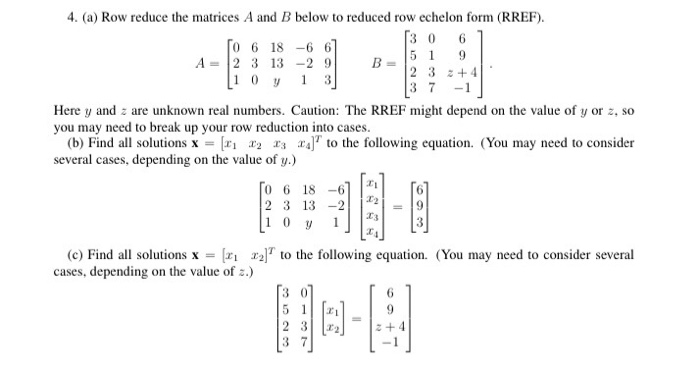4. (a) Row reduce the matrices A and B below to reduced row echelon form (RREF). (3 0 6 TO 6 18 -6 67 5 1 9 A = 2 3 13 -2 9 2 3 +41 11 0 y 13 37 -1 Here y and are unknown real numbers. Caution: The RREF might depend on the value of y or 2, so you may need to break up your row reduction into cases. (b) Find all solutions x =...

• ### Question 3 please answer clearly. A matrix A and its reduced row echelon form are given...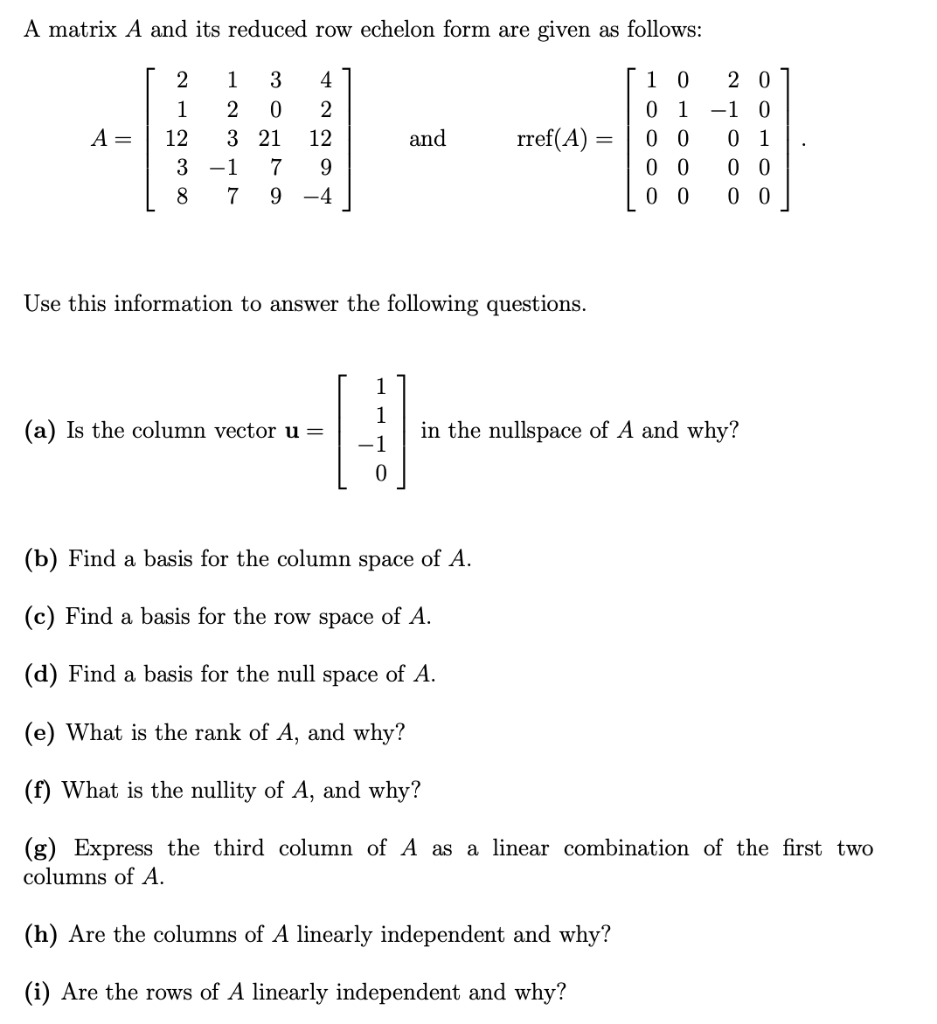Question 3 please answer clearly. A matrix A and its reduced row echelon form are given as follows: [ 2 1 3 41 | 1 2 0 2 A= 3 21 12 | 3 -1 7 9 18 7 9 -4 and rref(A) = [ 1 0 201 0 1 -1 0 0 0 0 1 0 0 0 0 | 0 0 0 0 Use this information to answer the following questions. (a) Is the column vector u= in...

• ### Consider the matrix A and the reduced row echelon form of A. A 1 -2 -2...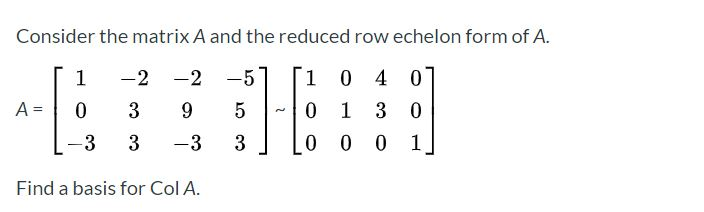Consider the matrix A and the reduced row echelon form of A. A 1 -2 -2 0 39 -3 3 -3 1 0 4 0 0 1 3 0 0 0 0 1 Find a basis for Col A.